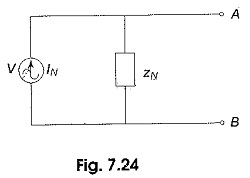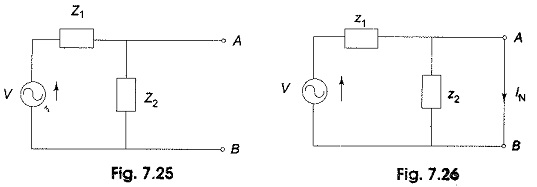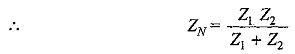## Norton Equivalent Circuit:

Another method of analysing a complex impedance circuit is given by Norton’s theorem. The Norton Equivalent Circuit form of any complex impedance circuit consists of an equivalent current source IN and an equivalent impedance ZN, arranged as shown in Fig. 7.24. The values of equivalent current and impedance depend on the values in the original circuit.Though Norton Equivalent Circuit is not the same as its original circuit, the output voltage and current are the same in both cases; Norton’s current is equal to the current passing through the short circuited output terminals and the value of impedance is equal to the impedance seen into the network across the output terminals.

Consider the circuit shown in Fig. 7.25.Norton’s equivalent for the circuit shown in Fig. 7.25 between points A and B is found as follows. The current passing through points A and B when it is short-circuited is the Norton’s equivalent current, as shown in Fig. 7.26.

Norton’s current IN = V/Z1

The impedance between points A and B, with the source replaced by a short circuit, is Norton’s equivalent impedance.

In Fig. 7.25, the impedance from A to B, Z2 is in parallel with Z1.Norton’s equivalent circuit is shown in Fig 7.27

Scroll to Top